### Home > INT3 > Chapter 8 > Lesson 8.1.1 > Problem8-17

8-17.
1.Given that log(2) ≈ 0.3010, log(3) ≈ 0.4771, and log(5) ≈ 0.6990, calculate each of the following logarithms without using the log button on your calculator. Homework Help ✎

1. log(6)

2. log(15)

3. log(9)

4. log(50)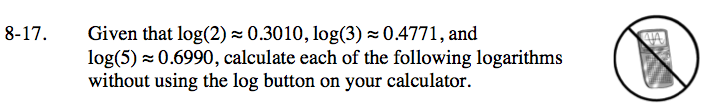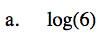log(6) = log (2 · 3)
Now use the properties of logarithms to expand.

log (2) + log (3) ≈ 0.7781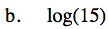Express 15 as a product of numbers whose logs are given. Then see the step in part (a).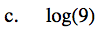log(9) can be rewritten as log(3 · 3) or log(32). Try both.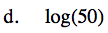50 = 2 · 52